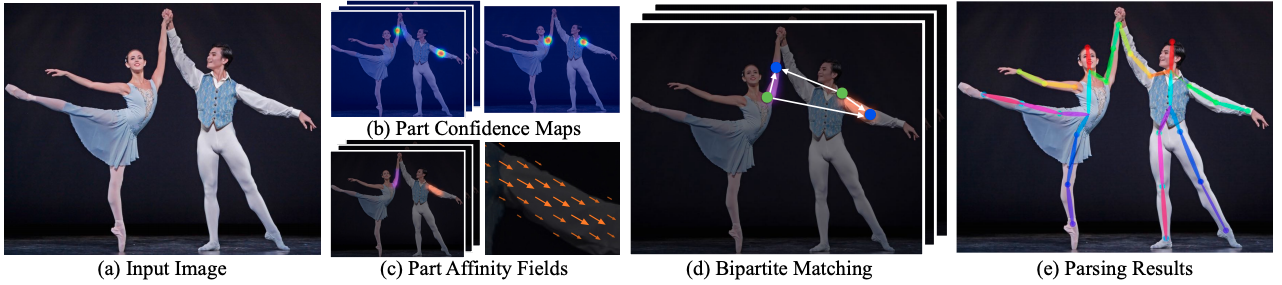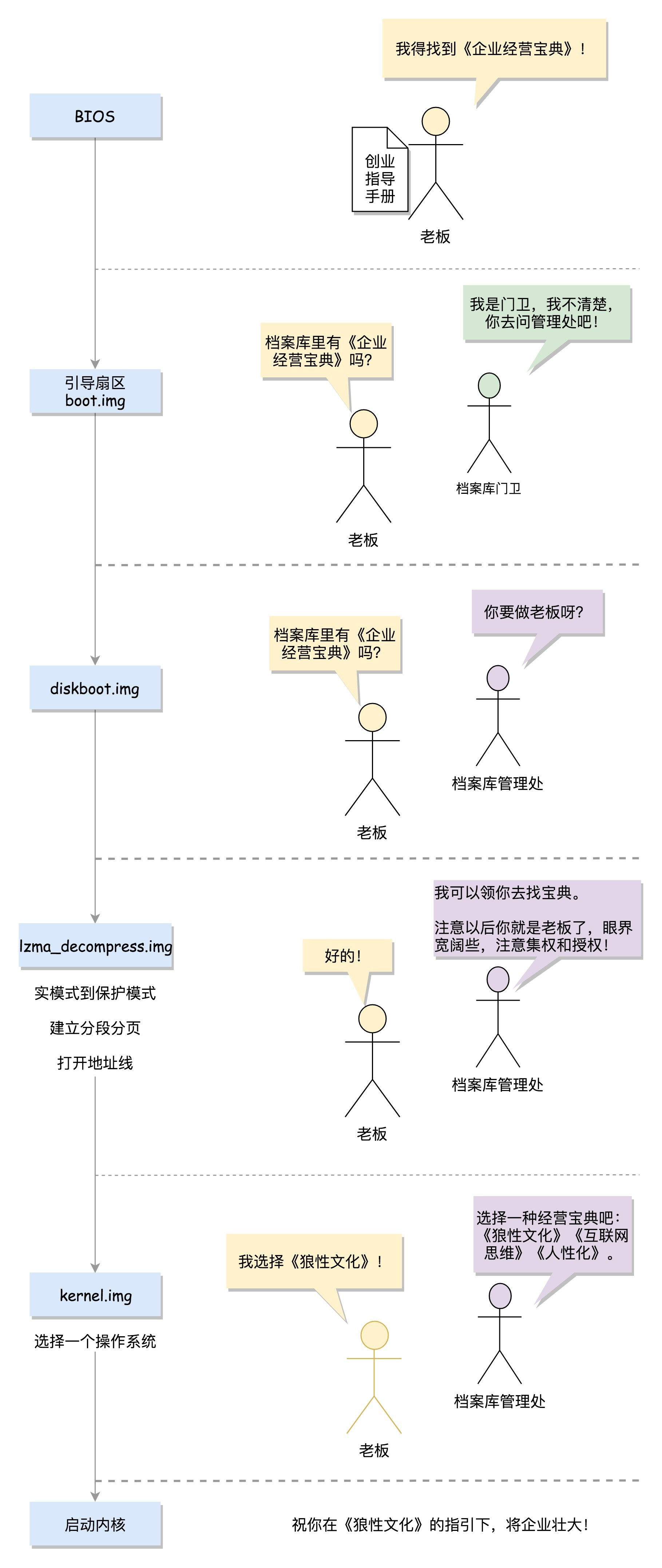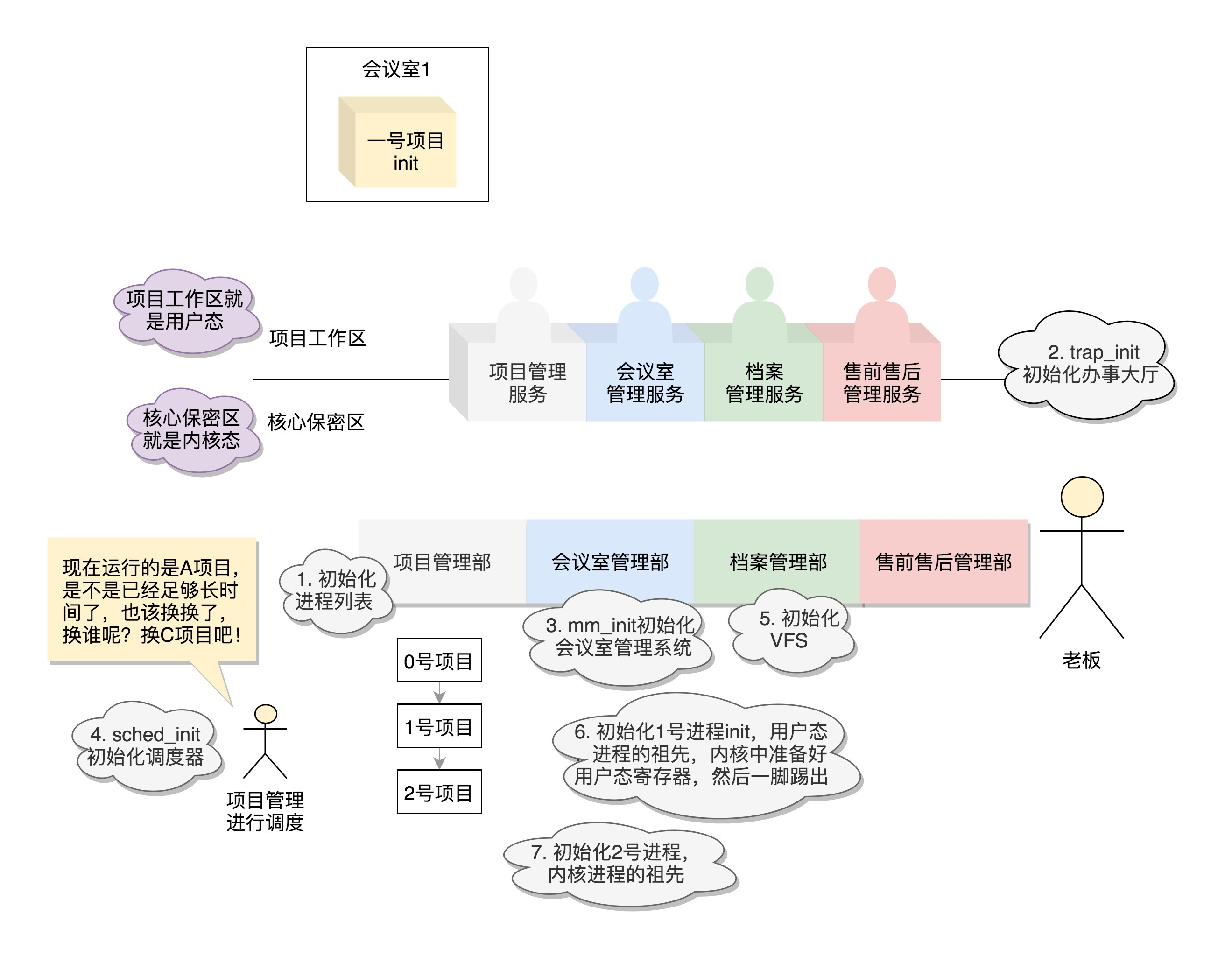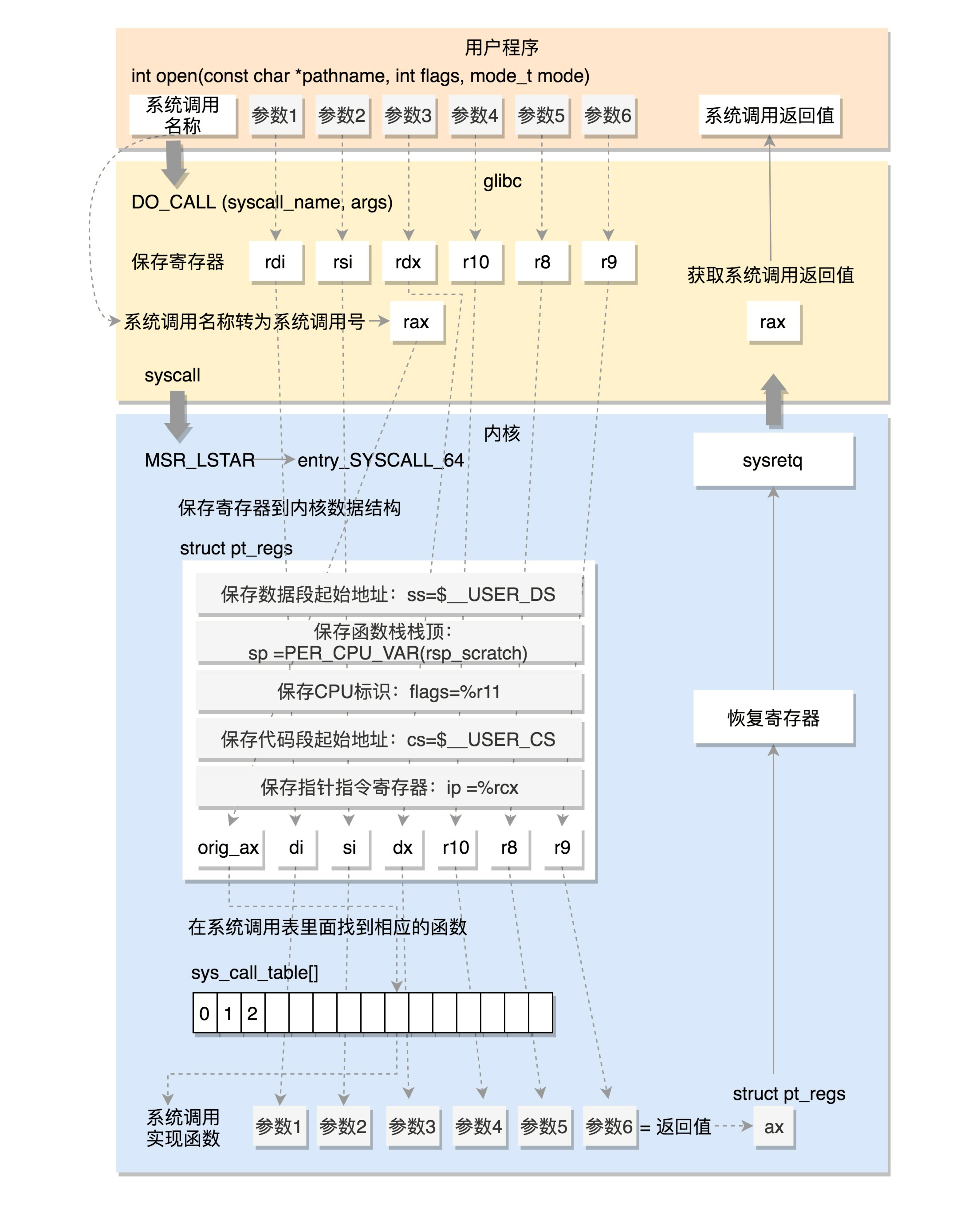# ARTS 打卡 -04Geek_yansheng25# Algorithm

## Question: Single Number

Given a non-empty array of integers, every element appears twice except for one. Find that single one.

class Solution {public:    int singleNumber(vector<int>& nums) {        std::sort(nums.begin(), nums.end());        int len = nums.size();        int res = 0;        for (int i = 0; i<len; i=i+2) {            if ((i + 1 >= len) || (nums[i+1] != nums[i])) {                res = nums[i];                break;            }        }        return res;    }};

Performance:

Memory usage beats 54.93%. Runtime beats 19.30%.

Use bit-wise XOR operation, "^".

class Solution {public:    int singleNumber(vector<int>& nums) {        int result=0;        for(int i=0;i<nums.size();i++)            result^=nums[i];        return result;            }};

# Review

## OpenCV

OpenCV (Open Source Computer Vision Library: http://opencv.org) is an open-source BSD-licensed library that includes several hundreds of computer vision algorithms.

OpenCV has a modular structure, which means that the package includes several shared or static libraries. The following modules are available:

• Core functionality (core) - a compact module defining basic data structures, including the dense multi-dimensional array Mat and basic functions used by all other modules.

• Image Processing (imgproc) - an image processing module that includes linear and non-linear image filtering, geometrical image transformations (resize, affine and perspective warping, generic table-based remapping), color space conversion, histograms, and so on.

• Video Analysis (video) - a video analysis module that includes motion estimation, background subtraction, and object tracking algorithms.

• Camera Calibration and 3D Reconstruction (calib3d) - basic multiple-view geometry algorithms, single and stereo camera calibration, object pose estimation, stereo correspondence algorithms, and elements of 3D reconstruction.

• 2D Features Framework (features2d) - salient feature detectors, descriptors, and descriptor matchers.

• Object Detection (objdetect) - detection of objects and instances of the predefined classes (for example, faces, eyes, mugs, people, cars, and so on).

• High-level GUI (highgui) - an easy-to-use interface to simple UI capabilities.

• Video I/O (videoio) - an easy-to-use interface to video capturing and video codecs.

• ... some other helper modules, such as FLANN and Google test wrappers, Python bindings, and others.

# Tips## 《08-内核初始化》

• 各个职能部门的创建；

• 用户态祖先进程的创建；

• 内核态祖先进程的创建。## 《09-系统调用》

64位系统调用的过程：# Share

OpenPose, developed by researchers at the Carnegie Mellon University can be considered as the state of the art approach for real-time human pose estimation

https://www.learnopencv.com/multi-person-pose-estimation-in-opencv-using-openpose/

https://medium.com/analytics-vidhya/understanding-openpose-with-code-reference-part-1-b515ba0bbc73

## OpenPose Architecture

The model takes as input a color image of size h x w and produces, as output, an array of matrices which consists of the confidence maps of Keypoints and Part Affinity Heatmaps for each keypoint pair.The above network architecture consists of two stages as explained below:

1. Stage 0: The first 10 layers of the VGGNet are used to create feature maps for the input image.

2. Stage 1: A 2-branch multi-stage CNN is used where

• The first branch predicts a set of 2D Confidence Maps (S) of body part locations ( e.g. elbow, knee etc.). A Confidence Map is a grayscale image which has a high value at locations where the likelihood of a certain body part is high. For example, the Confidence Map for the Left Shoulder is shown in Figure 2 below. It has high values at all locations where the there is a left shoulder.For the 18 point model, the first 19 matrices of the output correspond to the Confidence Maps.

• The second branch predicts a set of 2D vector fields (L) of Part Affinities (PAF), which encode the degree of association between parts (keypoints). The 20th to 57th matrices are the PAF matrices. In the figure below – part affinity between the Neck and Left shoulder is shown. Notice there is a large affinity between parts belonging to the same person.

The Confidence Maps are used to find the keypoints and the Affinity Maps are used to get the valid connections between the keypoints.## 评论